Functions and CALL Routines

# PROBMC Function

Returns a probability or a quantile from various distributions for multiple comparisons of means.
 Category: Probability

## Syntax

 PROBMC(distribution, q, prob, df, nparms<, parameters>)

### Arguments

distribution

is a character constant, variable, or expression that identifies the distribution. The following are valid distributions:

Distribution Argument
Analysis of Means
`ANOM`

One-sided Dunnett
`DUNNETT1`
Two-sided Dunnett
`DUNNETT2`
Maximum Modulus
`MAXMOD`
Partitioned Range
`PARTRANGE`

Studentized Range
`RANGE`
Williams
`WILLIAMS`

q

is the quantile from the distribution.

 Restriction: Either q or prob can be specified, but not both.
prob

is the left probability from the distribution.

 Restriction: Either prob or q can be specified, but not both.
df

is the degrees of freedom.

Note:   A missing value is interpreted as an infinite value.nparms

is the number of treatments.

Note:   For DUNNETT1 and DUNNETT2, the control group is not counted.parameters

is an optional set of nparms parameters that must be specified to handle the case of unequal sample sizes. The meaning of parameters depends on the value of distribution. If parameters is not specified, equal sample sizes are assumed, which is usually the case for a null hypothesis.

The PROBMC function returns the probability or the quantile from various distributions with finite and infinite degrees of freedom for the variance estimate.

The prob argument is the probability that the random variable is less than q. Therefore, p-values can be computed as 1- prob. For example, to compute the critical value for a 5% significance level, set prob= 0.95. The precision of the computed probability is O(10--8) (absolute error); the precision of computed quantile is O(10--5).

Note:   The studentized range is not computed for finite degrees of freedom and unequal sample sizes.Note:   Williams' test is computed only for equal sample sizes.### Formulas and Parameters

The equations listed here define expressions used in equations that relate the probability, prob, and the quantile, q, for different distributions and different situations within each distribution. For these equations, let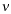be the degrees of freedom, df.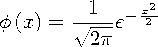### Computing the Analysis of Means

Analysis of Means (ANOM) applies to data that is organized as k (Gaussian) samples, the ith sample being of size ni. Let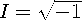. The distribution function [1, 2, 3, 4, 5] is the CDF for the maximum absolute of a k-dimensional multivariate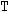vector, with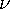degrees of freedom, and an associated correlation matrix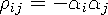. This equation can be written as
where
where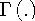,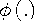, and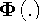, are the gamma function, the density, and the CDF from the standard normal distribution, respectively.

For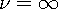, the distribution reduces to:
where
For the balanced case, the distribution reduces to:
where
and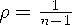The syntax for this distribution is:

 x=probmc('anom', q,p,nu,n,);

where

 x is a numeric value with the returned result. q is a numeric value denoting the quantile. p is a numeric value denoting the probability. One of p and q must be missing. nu is a numeric value denoting the degrees of freedom. n is a numeric value denoting the number of samples. alphai, i=1,...,k are optional numeric values denoting the alpha values from the first equation of this distribution (see Computing the Analysis of Means.

### Many-One t-Statistics: Dunnett's One-Sided Test

• This case relates the probability, prob, and the quantile, q, for the unequal case with finite degrees of freedom. The parameters are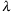1, ...,k, the value of nparms is set to k, and the value of df is set to. The equation follows:
• This case relates the probability, prob, and the quantile, q, for the equal case with finite degrees of freedom. No parameters are passed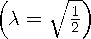, the value of nparms is set to k, and the value of df is set to. The equation follows:
• This case relates the probability, prob, and the quantile, q, for the unequal case with infinite degrees of freedom. The parameters are1, ...,k, the value of nparms is set to k, and the value of df is set to missing. The equation follows:
• This case relates the probability, prob, and the quantile, q, for the equal case with infinite degrees of freedom. No parameters are passed, the value of nparms is set to k, and the value of df is set to missing. The equation follows:
### Many-One t-Statistics: Dunnett's Two-sided Test

• This case relates the probability, prob, and the quantile, q, for the unequal case with finite degrees of freedom. The parameters are1, ...,k, the value of nparms is set to k, and the value of df is set to. The equation follows:
• This case relates the probability, prob, and the quantile, q, for the equal case with finite degrees of freedom. No parameters are passed, the value of nparms is set to k, and the value of df is set to. The equation follows:
• This case relates the probability, prob, and the quantile, q, for the unequal case with infinite degrees of freedom. The parameters are1, ...,k, the value of nparms is set to k, and the value of df is set to missing. The equation follows:
• This case relates the probability, prob, and the quantile, q, for the equal case with infinite degrees of freedom. No parameters are passed, the value of nparms is set to k, and the value of df is set to missing. The equation follows:
### Computing the Partitioned Range

RANGE applies to the distribution of the studentized range for n group means. PARTRANGE applies to the distribution of the partitioned studentized range. Let the n groups be partitioned into k subsets of size n1+ ...+ nk= n. Then the partitioned range is the maximum of the studentized ranges in the respective subsets, with the studentization factor being the same in all cases.
The syntax for this distribution is:

 x=probmc('partrange', q,p,nu,k,n1,...,nk);

where

 x is a numeric value with the returned result (either the probability or the quantile). q is a numeric value denoting the quantile. p is a numeric value denoting the probability. One of p and q must be missing. nu is a numeric value denoting the degrees of freedom. k is a numeric value denoting the number of groups. ni i=1,...,k are optional numeric values denoting the n values from the equation in this distribution (see Computing the Partitioned Range.

### The Studentized Range

Note:    The studentized range is not computed for finite degrees of freedom and unequal sample sizes.• This case relates the probability, prob, and the quantile, q, for the equal case with finite degrees of freedom. No parameters are passed, the value of nparms is set to k, and the value of df is set to. The equation follows:
• This case relates the probability, prob, and the quantile, q, for the unequal case with infinite degrees of freedom. The parameters are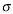1, ...,k, the value of nparms is set to k, and the value of df is set to missing. The equation follows:
• This case relates the probability, prob, and the quantile, q, for the equal case with infinite degrees of freedom. No parameters are passed, the value of nparms is set to k, and the value of df is set to missing. The equation follows:
### The Studentized Maximum Modulus

• This case relates the probability, prob, and the quantile, q, for the unequal case with finite degrees of freedom. The parameters are1, ...,k, the value of nparms is set to k, and the value of df is set to. The equation follows:
• This case relates the probability, prob, and the quantile, q, for the equal case with finite degrees of freedom. No parameters are passed, the value of nparms is set to k, and the value of df is set to. The equation follows:
• This case relates the probability, prob, and the quantile, q, for the unequal case with infinite degrees of freedom. The parameters are1, ...,k, the value of nparms is set to k, and the value of df is set to missing. The equation follows:
• This case relates the probability, prob, and the quantile, q, for the equal case with infinite degrees of freedom. No parameters are passed, the value of nparms is set to k, and the value of df is set to missing. The equation follows:
### Williams' Test

PROBMC computes the probabilities or quantiles from the distribution defined in Williams (1971, 1972) (See References). It arises when you compare the dose treatment means with a control mean to determine the lowest effective dose of treatment.

Note:   Williams' Test is computed only for equal sample sizes.Let X1, X2, ..., Xk be identical independent N(0,1) random variables. Let Yk denote their average given by
It is required to compute the distribution of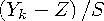where

 Yk is as defined previously Z is an N(0,1) independent random variable S is such that ½S2 is a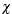2 variable withdegrees of freedom.

As described in Williams (1971) (See References), the full computation is extremely lengthy and is carried out in three stages.

1. Compute the distribution of Yk. It is the fundamental (expensive) part of this operation and it can be used to find both the density and the probability of Yk. Let Ui be defined as
You can write a recursive expression for the probability of Yk > d, with d being any real number.
To compute this probability, start from an N(0,1) density function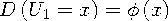and recursively compute the convolution
From this sequential convolution, it is possible to compute all the elements of the recursive equation for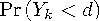, shown previously.

2. Compute the distribution of Yk - Z. This computation involves another convolution to compute the probability
3. Compute the distribution of (Yk - Z)/S. This computation involves another convolution to compute the probability
The third stage is not needed when=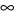. Due to the complexity of the operations, this lengthy algorithm is replaced by a much faster one when k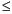15 for both finite and infinite degrees of freedom. For k16, the lengthy computation is carried out. It is extremely expensive and very slow due to the complexity of the algorithm.

The MEANS statement in the GLM Procedure of SAS/STAT Software computes the following tests:

• Dunnett's one-sided test

• Dunnett's two-sided test

• Studentized Range

### Example 1: Computing Probabilities by Using PROBMC

This example shows how to compute probabilities.

```data probs;
array par{5};
par{1}=.5;
par{2}=.51;
par{3}=.55;
par{4}=.45;
par{5}=.2;
df=40;
q=1;
do test="dunnett1","dunnett2", "maxmod";
prob=probmc(test, q, ., df, 5, of par1-par5);
put test \$10. df q e18.13 prob e18.13;
end;
run; ```

SAS writes the following results to the log:

```DUNNETT1  40  1.00000000000E+00 4.82992196083E-01
DUNNETT2  40  1.00000000000E+00 1.64023105316E-01
MAXMOD    40  1.00000000000E+00 8.02784203408E-01```

### Example 2: Computing the Analysis of Means

```data _null_;
q1=probmc('anom',.,0.9,.,20);                      put q1=;
q2=probmc('anom',.,0.9,20,5,0.1,0.1,0.1,0.1,0.1);  put q2=;
q3=probmc('anom',.,0.9,20,5,0.5,0.5,0.5,0.5,0.5);  put q3=;
q4=probmc('anom',.,0.9,20,5,0.1,0.2,0.3,0.4,0.5);  put q4=;
run;```

SAS writes the following output to the log:

```q1=2.7892895753
q2=2.4549773558
q3=2.4549773558
q4=2.4532130238```

### Example 3: Comparing Means

This example shows how to compare group means to find where the significant differences lie. The data for this example is taken from a paper by Duncan (1955) (See References) and can also be found in Hochberg and Tamhane (1987) (See References).

The following values are the group means:

 49.6 71.2 67.6 61.5 71.3 58.1 61
For this data, the mean square error is s2 = 79.64 (s = 8.924) with= 30.
```data duncan;
array tr{7}\$;
array mu{7};
n=7;
do i=1 to n;
input tr{i} \$1. mu{i};
end;
input df s alpha;
prob= 1-alpha;
/* compute the interval */
x = probmc("RANGE", ., prob, df, 7);
w = x * s / sqrt(6);
/* compare the means */
do i = 1 to n;
do j = i + 1 to n;
dmean = abs(mu{i} - mu{j});
if dmean >= w then do;
put tr{i} tr{j} dmean;
end;
end;
end;
datalines;
A 49.6
B 71.2
C 67.6
D 61.5
E 71.3
F 58.1
G 61.0
30 8.924 .05
;
run;```

SAS writes the following output to the log:

```A B 21.6
A C 18
A E 21.7```

### Example 4: Computing the Partitioned Range

```data _null_;
q1=probmc('partrange',.,0.9,.,4,3,4,5,6);  put q1=;
q2=probmc('partrange',.,0.9,12,4,3,4,5,6); put q2=;
run;```

SAS writes the following output to the log:

```q1=4.1022395729
q2=4.788862411```

### Example 5: Computing Confidence Intervals

This example shows how to compute 95% one-sided and two-sided confidence intervals of Dunnett's test. This example and the data come from Dunnett (1955) (See References) and can also be found in Hochberg and Tamhane (1987) (See References). The data are blood count measurements on three groups of animals. As shown in the following table, the third group serves as the control, while the first two groups were treated with different drugs. The numbers of animals in these three groups are unequal.

Treatment Group: Drug A Drug B Control

9.76 12.80 7.40

8.80 9.68 8.50

7.68 12.16 7.20

9.36 9.20 8.24

10.55 9.84

8.32
Group Mean 8.90 10.88 8.25
n 4 5 6

The mean square error s2 = 1.3805 (s = 1.175) with= 12.

```data a;
array drug{3}\$;
array count{3};
array mu{3};
array lambda{2};
array delta{2};
array left{2};
array right{2};

/* input the table */
do i = 1 to 3;
input drug{i} count{i} mu{i};
end;

/* input the alpha level,    */
/* the degrees of freedom,   */
/* and the mean square error */
input alpha df s;

/* from the sample size, */
/* compute the lambdas   */
do i = 1 to 2;
lambda{i} = sqrt(count{i}/
(count{i} + count{3}));
end;

/* run the one-sided Dunnett's test */
test="dunnett1";
x = probmc(test, ., 1 - alpha, df,
2, of lambda1-lambda2);
do i = 1 to 2;
delta{i} = x * s *
sqrt(1/count{i} + 1/count{3});
left{i} = mu{i} - mu{3} - delta{i};
end;
put test \$10. x left{1} left{2};

/* run the two-sided Dunnett's test */
test="dunnett2";
x = probmc(test, ., 1 - alpha, df,
2, of lambda1-lambda2);
do i=1 to 2;
delta{i} = x * s *
sqrt(1/count{i} + 1/count{3});
left{i} = mu{i} - mu{3} - delta{i};
right{i} = mu{i} - mu{3} + delta{i};
end;
put test \$10. left{1} right{1};
put test \$10. left{2} right{2};
datalines;
A 4 8.90
B 5 10.88
C 6 8.25
0.05 12 1.175
;
run;```

SAS writes the following output to the log:

```DUNNETT1  2.1210786586 -0.958751705 1.1208571303
DUNNETT2  -1.256411895 2.5564118953
DUNNETT2  0.8416271203 4.4183728797```

### Example 6: Computing Williams' Test

In the following example, a substance has been tested at seven levels in a randomized block design of eight blocks. The observed treatment means are as follows:

Treatment Mean
X0 10.4
X1 9.9
X2 10.0
X3 10.6
X4 11.4
X5 11.9
X6 11.7

The mean square, with (7 - 1)(8 - 1) = 42 degrees of freedom, is s2 = 1.16.

Determine the maximum likelihood estimates Mi through the averaging process.

• Because X0 > X1, form X0,1 = (X0 + X1)/2 = 10.15.

• Because X0,1 > X2, form X0,1,2 = (X0 + X1 + X2)/3 = (2X0,1 + X2)/3 = 10.1.

• X0,1,2 < X3 < X4 < X5

• Because X5 > X6, form X5,6 = (X5 + X6)/2 = 11.8.

Now the order restriction is satisfied.

The maximum likelihood estimates under the alternative hypothesis are:

 M0 = M1 = M2 = X0,1,2 = 10.1 M3 = X3 = 10.6 M4 = X4 = 11.4 M5 = M6 = X5,6 = 11.8

Now compute
, and the probability that corresponds to k = 6,= 42, and t = 2.60 is .9924467341, which shows strong evidence that there is a response to the substance. You can also compute the quantiles for the upper 5% and 1% tails, as shown in the following table.

SAS Statements Results
`prob=probmc("williams",2.6,.,42,6);`
`0.99244673`
`quant5=probmc("williams",.,.95,42,6);`
`1.80654052`
`quant1=probmc("williams",.,.99,42,6);`
`2.49087829`

 Functions:

 Guirguis, G. H. and R. D. Tobias. 2004. "On the computation of the distribution for the analysis of means." Communications in Statistics: Simulation and Computation 33: 861-887. Nelson, P. R. 1981. "Numerical evaluation of an equicorrelated multivariate non-central t distribution." Communications in Statistics: Part B - Simulation and Computation 10: 41-50. Nelson, P. R. 1982. "Exact critical points for the analysis of means." Communications in Statistics: Part A - Theory and Methods 11: 699-709. Nelson, P. R. 1982a. "An Approximation for the Complex Normal Probability Integral." BIT 22(1): 94-100. Nelson, P. R. 1988. "Application of the analysis of means." Proceedings of the SAS Users Group International Conference 13: 225-230. Nelson, P. R. 1991. "Numerical evaluation of multivariate normal integrals with correlations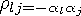." The Frontiers of Statistical Scientific Theory and Industrial Applications 2: 97-114. Nelson, P. R. 1993. "Additional Uses for the Analysis of Means and Extended Tables of Critical Values." Technometrics 35: 61-71.Previous Page | Next Page | Top of Page